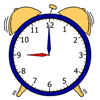#### You may also like### Triangle Animals

How many different ways can you find to join three equilateral triangles together? Can you convince us that you have found them all?### Triple Cubes

This challenge involves eight three-cube models made from interlocking cubes. Investigate different ways of putting the models together then compare your constructions.### Order, Order!

Can you place these quantities in order from smallest to largest?

# The Add and Take-away Path

##### Age 5 to 7Challenge Level

Jack from Heacham Infant and Nursery School looked at Thea's and William's routes and told us:

The score each time was $16$.
It did not matter which route you take, the score was the same.

Ellie from Short Wood Primary School looked at the red and blue routes.  She said:

the red path takes the route of:
minus $1$, plus $2$, plus $2$ , plus $1$, plus $1$, plus $1$, plus $1$, plus $2$, minus $1$ which will give you a total score of $18$ points

the blue path takes the route of:
plus $2$, plus $1$, plus $2$, plus $2$, plus $1$ which will give you the score of $18$ points

So the solution is that if you take the longer red path you will score exactly the same as the shorter blue path.

David from Belmont Primary School began to explain why these two routes gave the same total:

The reason that it is impossible to get any other number than $18$ is because the shortest path from start to finish is $2$ right and $3$ upwards, leaving you with $18$ ($10+1+1+2+2+2$). Therefore, any other move to attempt getting less points by subtracting (moving left/down) would be met by an equal addition of the same number (moving right/upward). So ultimately any path (no matter how complex) would lead to $18$.

Someome from St Matthew's gave a good explanation too:

I conjecture that the line you draw can be of any length.
The quickest way to get from start to finish is in $5$ moves and this adds up to $18$ (starting with $10$).
If you imagine that your line is a brick wall and you make an extension in the middle of it, then as you move backwards and forwards, the operations always cancel each other out. E.g. if you move up $1$, you add $2$ and then if you move down $1$, you take away $2$. Whatever shapes you make it doesn't matter because the moves always cancel each other out.

Well done!